# 12 Best Calculator Apps for Android | 2021

The calculator is a utility application that everyone uses in their day to day life, be it a business person or a student. Generally, for different functions, we need to install multiple applications on our android device. Students need scientific calculators, algebraic calculators, geometric calculators, maths calculators, and many others. On the other hand, working people need business calculators, marketing calculators, finance calculators, and currency converters.

Also see: Best Ringtone cutter apps

Having said this, let’s take a look at some of the best calculator apps which you can get to get your calculations to go easy! For basic mathematical operations, one can use the default calculators which are installed in every device. Here is the list of best calculator apps for Android.

## Best Calculator Apps for Android

Here is the list of best calculator apps for android:

### 1. All in one calculator by Mindbox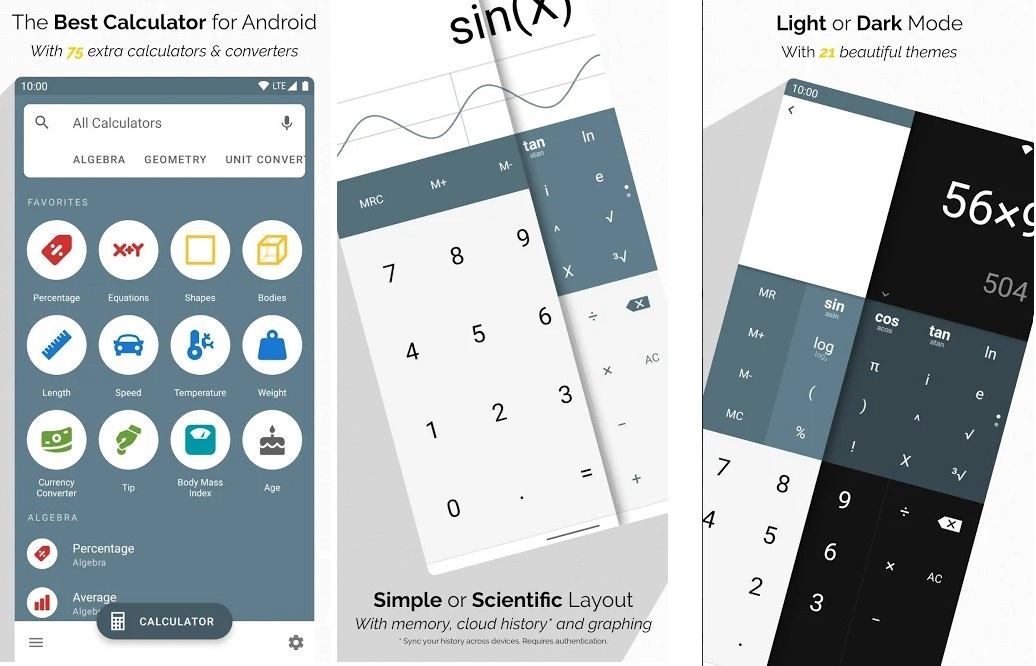It is a free calculator app for Android featuring over 75+ calculators and unit converters categorized under percentage, shapes, finance, currency converter, Geometry, Unit Converters, Finance, Health, and more. The standard calculator includes percent key, negative sign, brackets, and history viewer whereas the advanced mode includes memory keys and functions for trigonometry, roots, exponents, and logarithm found in scientific calculators.

The unit converter supports Energy, temperature, length, weight, area, volume, Speed, and many more measurement units along with a currency converter. The financial one includes interest calculators using simple and compound interest along with a savings calculator and planner. The fractions/percentage calculator features basic functions.

Health calc measures BMI, BMR, body fat percentage, and calorie calculators to help you keep track of your body weight.

### 2. Photomath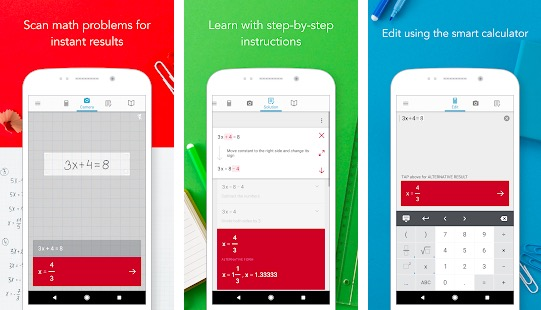PhotoMath is an app that utilizes your phone camera to solve maths problems. It works only with the printed text, it won’t be solving your scribbles. Handles math problems that include fractions, decimal numbers, linear equations, and several functions like logarithms.

Photomath is free and works without wi-fi or data. So, Photomath is always available whether you’re on the bus, on a trip, or just offline.

Also see: Best Fake call apps for Android

### 3. Microsoft Math Solver: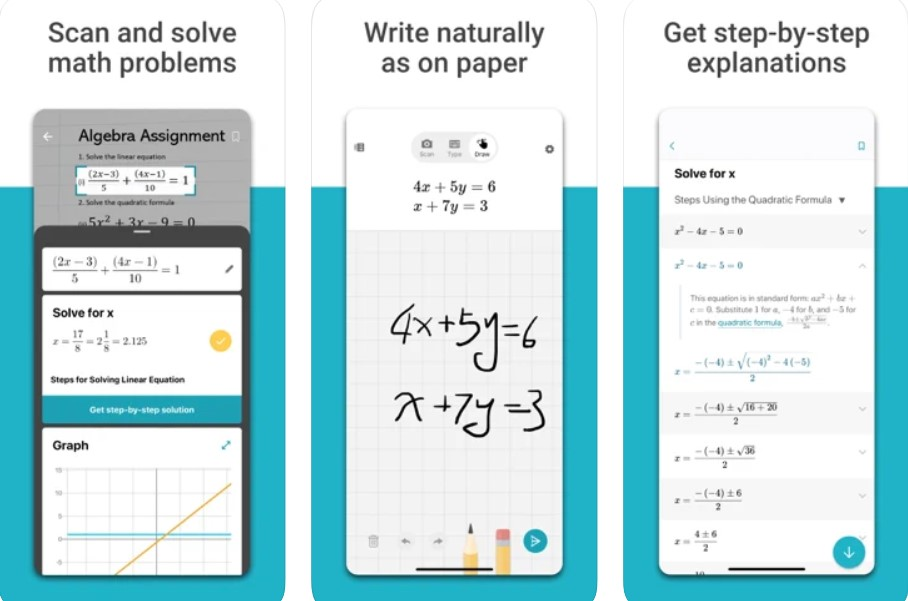The Microsoft math solver app gives help with a variety of problems including arithmetic, algebra, trigonometry, calculus, statistics, and other topics using an advanced AI-powered math solver. It instantly identifies the problem and helps you to solve it with Step-By-Step Explanations, and an interactive graph.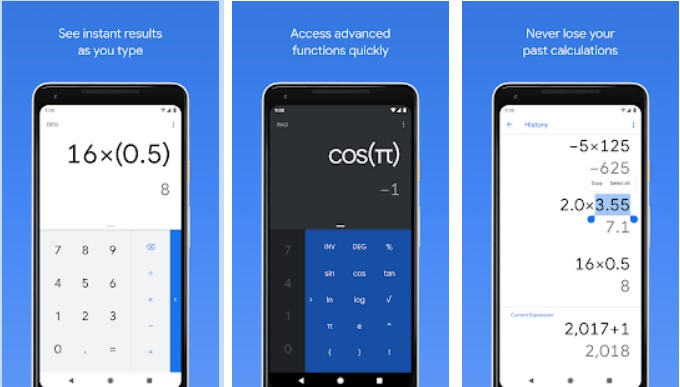The calculator by Google is the popular calculator that every android phone already has. It gives simple and advanced mathematical functions in a beautifully designed app. It performs basic calculations such as addition, subtraction, multiplication, and division and it can also do scientific operations such as trigonometric, logarithmic, and exponential functions.

### 5. MyScript Calculator 2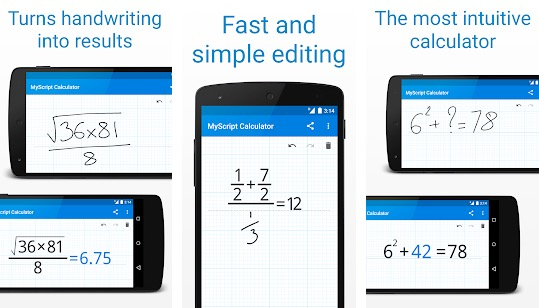It is an app for both iOS and Android that lets you perform mathematical operations with features like freehand writing of expressions on the touch screen. The screen looks like graph paper with features like undo, redo, and trash. It also features palm rejection- i.e. it ignores the touches of your palms, useful for those who rest their hands on the screen.

### 6. Clevcalc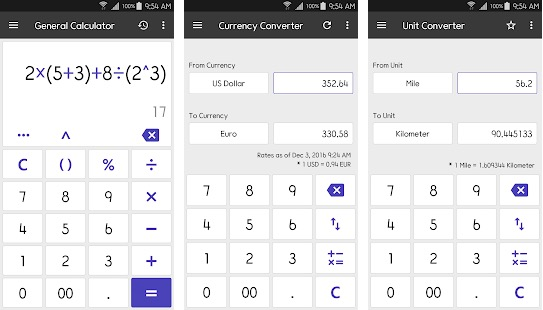This is also a multipurpose calculator app that can do lots of things. It can perform General calculations, Conversion of all units, Currency conversion, Calculation of the reduction, World clock conversion and GPA calculation.it also comes with an Ovulation calculator that calculates ovulation time and fertility using the menstrual cycle billing date. It can Calculate the unit price by entering the price and the amount. In addition, It can also do the calculation of health: BMI and BMR metabolism. It can also calculate energy efficiency to calculate fuel consumption for energy production.

### 7. Desmos Graphing CalculatorIt allows us to enter functions manually, explore preloaded functions, or download graphs from the server. To enter functions manually, users input values using the on-screen keyboard and then scroll and zoom onto the graph it creates. Users can manipulate variables by giving them value and watching the graph adjust. Features involve:

• Graphing: Plot polar, cartesian, or parametric graphs.
• Tables: Input and plot data or create an input-output table for any function
• Statistics: Find best-fit lines, parabolas, and more.
• Zooming: Scale the axes independently or at the same time with the pinch of two fingers or edit the window size manually to get the perfect window.
• Points of Interest: Touch a curve to show maximums, minimums, and points of intersection. Tap the grey points of interest to see their coordinates. Hold and drag along a curve to see the coordinates change under your finger.
• Inequalities: Plot cartesian and polar inequalities.

### 8. CalcKit All-in-One Calculator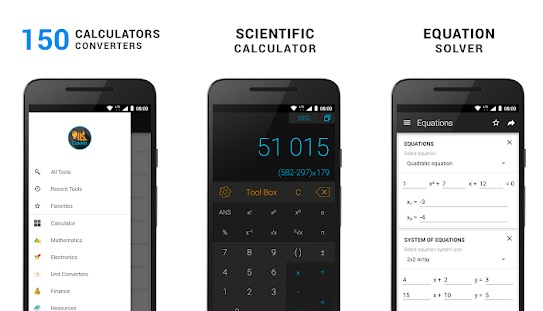CalcKit is another interesting app that offers everything you need from a calculator app. The app features over 150 calculators including unit converters, customizable Scientific Calculator, floating calculator widget, and more. You can use the app to save complex 3D geometry as well.

The app can be used to solve Geometry, Equations, Analytical Geometry, Algebra, Matrix, and Electronics problems. You can try several kinds of converters.

The app has an integrated notepad and integrated search. It also offers Light & Dark themes depending on your choice. It is also available in several languages, which makes it available for a wide range of people.

### 9. Calculator++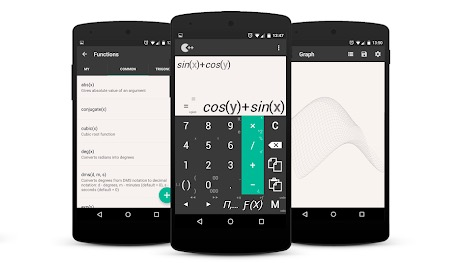Calculator++ is also a smart calculator app for Android. This app looks like Goole Calculator. It has a Standard layout along with an Engineer layout for more calculation options. The app includes gestures as well. It also has a floating window feature that lets you use the calculator over the top of other apps.

### 10. Scalar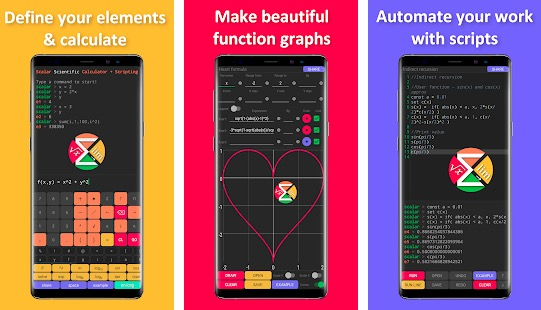Scalar is also one of the best calculator apps for Android. The app comes with a powerful math engine and math scripting language that lets users write scripts capable of working on complex things. This is an advanced app where you can create scripts. You can define arguments and functions along with using those functions and arguments in calculations, expressions, and graphs.

### 11. Scientific Calculator by CalcTasticScientific Calculator by CalcTastic is for those who need a calculator app for scientific calculations. It also has a basic calculator but is known for its scientific calculation options. You can use this app for solving complex problems. The app also comes with customizable theme options.

### 12. GeoGebra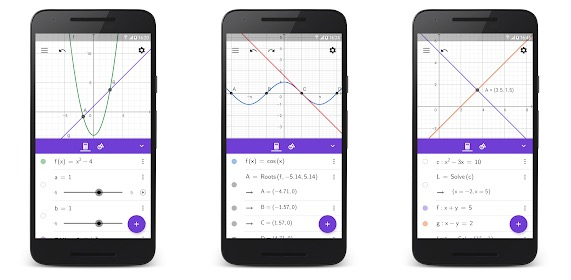GeoGebra app is basically for solving the graph functions. The app can do calculus, statistics, geometry, and algebra for you. It is an easy to use app that makes it easy to create graphs and shapes by dragging & dropping. The app also lets you check real-time changes in your calculations and variables. GeoGebra app lets you plot functions, polar and parametric curves. You can save and share your results with friends.